# Types of Axis in WinUI Chart (SfCartesianChart)

6 Dec 202212 minutes to read

Cartesian chart supports the following types of chart axis.

• NumericalAxis
• CategoryAxis
• DateTimeAxis

## Numerical Axis

NumericalAxis is used to plot numerical values to the chart. NumericalAxis can be added for both XAxes and YAxes.

``````<chart:SfCartesianChart>
. . .
<chart:SfCartesianChart.XAxes>
<chart:NumericalAxis/>
</chart:SfCartesianChart.XAxes>
<chart:SfCartesianChart.YAxes>
<chart:NumericalAxis/>
</chart:SfCartesianChart.YAxes>

</chart:SfCartesianChart>``````
``````SfCartesianChart chart = new SfCartesianChart();
. . .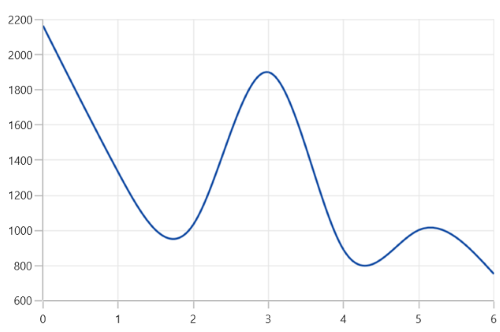### Interval

Axis interval can be customized by using the Interval property. By default, interval will be calculated based on the minimum and maximum value of the provided data.

``````<chart:SfCartesianChart>
. . .
<chart:SfCartesianChart.XAxes>
<chart:NumericalAxis Interval="10" />
</chart:SfCartesianChart.XAxes>
<chart:SfCartesianChart.YAxes>
<chart:NumericalAxis/>
</chart:SfCartesianChart.YAxes>

</chart:SfCartesianChart>``````
``````SfCartesianChart chart = new SfCartesianChart();
. . .
NumericalAxis primaryAxis = new NumericalAxis()
{
Interval = 10,
};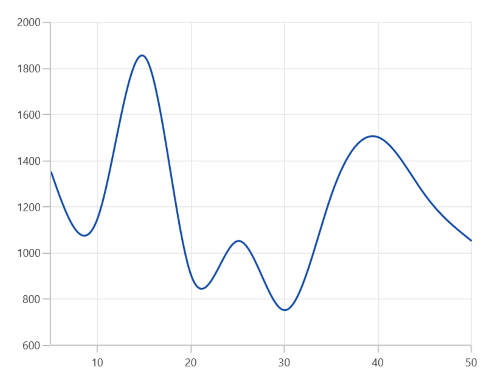### Customizing the Range

Maximum and Minimum properties of axis is used for setting the maximum and minimum value of the axis range respectively.

NOTE

If minimum or maximum value is set, the other value is calculated by default internally.

``````<chart:SfCartesianChart.YAxes>
<chart:NumericalAxis Maximum="2750"
Minimum="250"
Interval="250"/>
</chart:SfCartesianChart.YAxes>``````
``````NumericalAxis secondaryAxis = new NumericalAxis()
{
Maximum = 2750,
Minimum = 250,
Interval = 250
};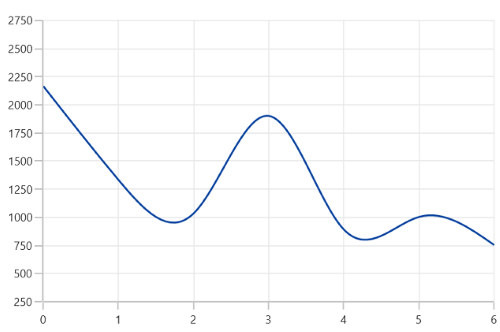## Category Axis

The CategoryAxis is an indexed based axis that plots values based on the index of the data point collection. The points are equally spaced here.

``````<chart:SfCartesianChart>
. . .
<chart:SfCartesianChart.XAxes>
<chart:CategoryAxis/>
</chart:SfCartesianChart.XAxes>

</chart:SfCartesianChart>``````
``````SfCartesianChart chart = new SfCartesianChart();
. . .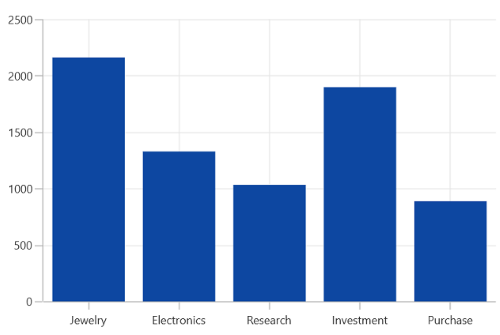### Label placement

The LabelPlacement property in CategoryAxis is used to placed the axis labels based on ticks and between ticks. The default value of LabelPlacement is OnTicks.

``````<chart:SfCartesianChart>
. . .
<chart:SfCartesianChart.XAxes>
<chart:CategoryAxis LabelPlacement="BetweenTicks"/>
</chart:SfCartesianChart.XAxes>

</chart:SfCartesianChart>``````
``````SfCartesianChart chart = new SfCartesianChart();
. . .
CategoryAxis primaryAxis = new CategoryAxis()
{
LabelPlacement = LabelPlacement.BetweenTicks
};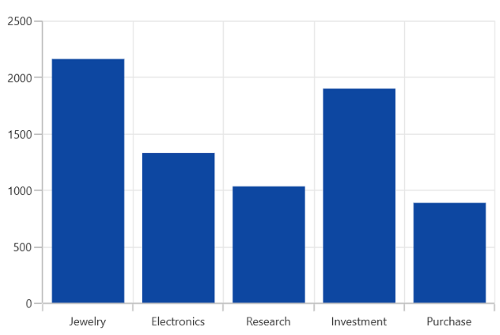### Interval

By default, the CategoryAxis axis labels are display with fixed interval 1. It can customized by using the Interval property of axis.

``````<chart:SfCartesianChart>
. . .
<chart:SfCartesianChart.XAxes>
<chart:CategoryAxis Interval="2" />
</chart:SfCartesianChart.XAxes>
<chart:SfCartesianChart.YAxes>
<chart:NumericalAxis/>
</chart:SfCartesianChart.YAxes>

</chart:SfCartesianChart>``````
``````SfCartesianChart chart = new SfCartesianChart();
. . .
CategoryAxis primaryAxis = new CategoryAxis()
{
Interval = 2,
};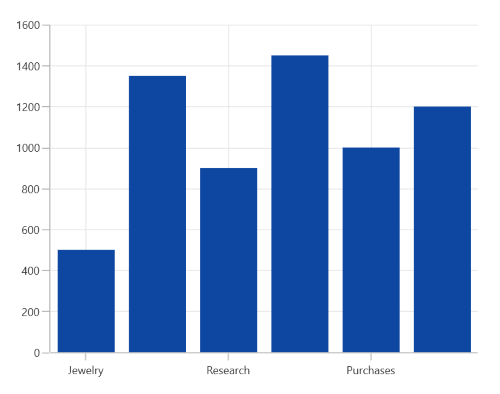## DateTime Axis

DateTimeAxis is used to plot `DateTime` values. The DateTimeAxis is widely used to make financial charts in places like the Stock Market, where index plotting is done every day.

``````<chart:SfCartesianChart>
. . .
<chart:SfCartesianChart.XAxes>
<chart:DateTimeAxis>
<chart:DateTimeAxis.LabelStyle>
<chart:LabelStyle LabelFormat="MMM-yy" />
</chart:DateTimeAxis.LabelStyle>
</chart:DateTimeAxis>
</chart:SfCartesianChart.XAxes>

</chart:SfCartesianChart>``````
``````SfCartesianChart chart = new SfCartesianChart();
. . .
DateTimeAxis primaryAxis = new DateTimeAxis()
{
LabelStyle = new LabelStyle() { LabelFormat= "MMM-yy" }
};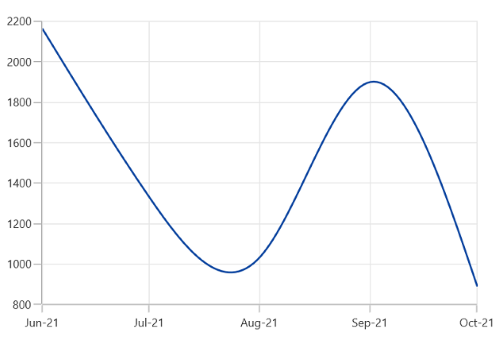### Interval

In DateTimeAxis, intervals can be customized by using the Interval and IntervalType properties. For example, setting Interval as 6 and IntervalType as Months will consider 6 months as interval.

``````<chart:SfCartesianChart>
. . .
<chart:SfCartesianChart.XAxes>
<chart:DateTimeAxis Interval="6"
IntervalType="Months" >
<chart:DateTimeAxis.LabelStyle>
<chart:LabelStyle LabelFormat="MMM-yy" />
</chart:DateTimeAxis.LabelStyle>
</chart:DateTimeAxis>
</chart:SfCartesianChart.XAxes>
<chart:SfCartesianChart.YAxes>
<chart:NumericalAxis/>
</chart:SfCartesianChart.YAxes>

</chart:SfCartesianChart>``````
``````SfCartesianChart chart = new SfCartesianChart();
. . .
DateTimeAxis primaryAxis = new DateTimeAxis()
{
Interval = 6,
IntervalType = DateTimeIntervalType.Months,
LabelStyle = new LabelStyle() { LabelFormat= "MMM-yy" }
};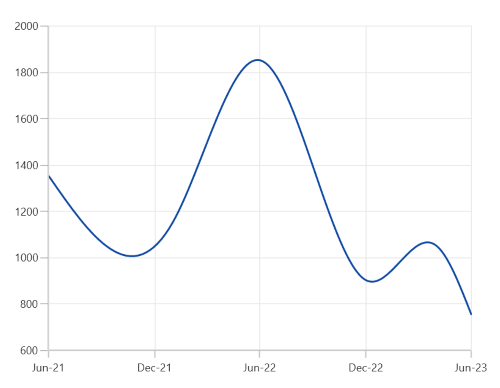### Customizing the Range

Minimum and Maximum properties behavior is same as in NumericalAxis instead of setting numerical value, have to set date time values.

``````<chart:SfCartesianChart>
. . .
<chart:SfCartesianChart.XAxes>
<chart:DateTimeAxis Minimum="2021/05/1"
Maximum="2021/11/01" >
<chart:DateTimeAxis.LabelStyle>
<chart:LabelStyle LabelFormat="MMM-yy" />
</chart:DateTimeAxis.LabelStyle>
</chart:DateTimeAxis>
</chart:SfCartesianChart.XAxes>

</chart:SfCartesianChart>``````
``````SfCartesianChart chart = new SfCartesianChart();
. . .
DateTimeAxis primaryAxis = new DateTimeAxis()
{
Minimum = new DateTime(2021,05,10),
Maximum = new DateTime(2021,11,01),
LabelStyle = new LabelStyle() { LabelFormat= "MMM-yy" }
};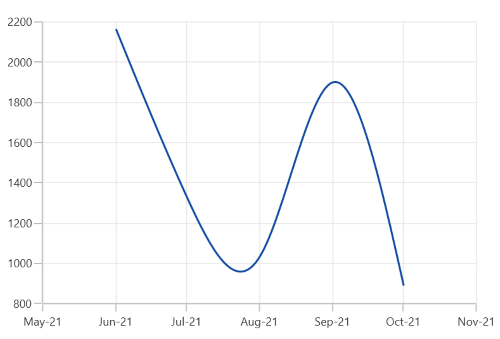## Inversed

Axis can be inverted by using the IsInversed property. The default value of this property is `False`.

``````<chart:SfCartesianChart>
. . .
<chart:SfCartesianChart.YAxes>
<chart:NumericalAxis IsInversed="True"/>
</chart:SfCartesianChart.YAxes>

</chart:SfCartesianChart>``````
``````SfCartesianChart chart = new SfCartesianChart();
. . .
NumericalAxis secondaryAxis = new NumericalAxis();
secondaryAxis.IsInversed = true;## Opposed Axis

Cartesian charts allow positioning the axis to opposite position by setting the OpposedPosition property. The default value of this property is `False.`

``````<chart:SfCartesianChart>
. . .
<chart:SfCartesianChart.YAxes>
<chart:NumericalAxis OpposedPosition="True"/>
</chart:SfCartesianChart.YAxes>

</chart:SfCartesianChart>``````
``````SfCartesianChart chart = new SfCartesianChart();
. . .
NumericalAxis secondaryAxis = new NumericalAxis();
secondaryAxis.OpposedPosition = true;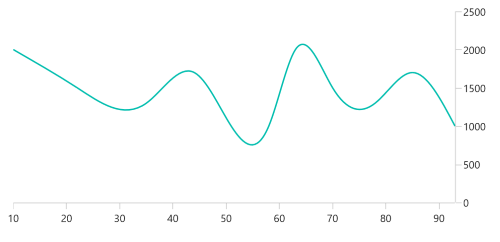## Multiple Axes

Cartesian charts provides support to arrange the multiple series inside the same chart area with specified x-axis and y-axis. There are two properties XAxisName and YAxisName in all the Cartesian series type, which is used to provide multiple axes support. These axes can be arranged in a stacking order or in a side by side pattern.

By default, all the series are plotted based on the 0th index axis of XAxes and YAxes.

``````<chart:SfCartesianChart>
. . .
<chart:SfCartesianChart.XAxes>
<chart:DateTimeAxis ShowMajorGridLines="False" />
</chart:SfCartesianChart.XAxes>

<chart:SfCartesianChart.YAxes>
<chart:NumericalAxis ShowMajorGridLines="False" />
<chart:NumericalAxis Name="Series_YAxis"
ShowMajorGridLines="False" />
</chart:SfCartesianChart.YAxes>

<chart:SfCartesianChart.Series>
<chart:SfCartesianChart.Series>
<chart:ColumnSeries ItemsSource="{Binding Data1}"
XBindingPath="Date"
YBindingPath="Value"
YAxisName="Series_YAxis">
</chart:ColumnSeries>
<chart:SplineSeries ItemsSource="{Binding Data}"
XBindingPath="Date"
YBindingPath="Value"/>
</chart:SfCartesianChart.Series>

</chart:SfCartesianChart>

</ContentPage>``````
``````SfCartesianChart chart = new SfCartesianChart();
. . .
DateTimeAxis primaryAxis = new DateTimeAxis()
{
ShowMajorGridLines = false
};

NumericalAxis secondaryAxis = new NumericalAxis()
{
ShowMajorGridLines = false
};
secondaryAxis.Name = "Series_YAxis";

NumericalAxis secondaryAxis1 = new NumericalAxis();

ColumnSeries series1 = new ColumnSeries()
{
YAxisName = "Series_YAxis"
ItemsSource = new ViewModel().Demands,
XBindingPath = "Demand",
YBindingPath = "Year2011"
};

SplineSeries series2 = new SplineSeries()
{
ItemsSource = new ViewModel().Demands,
XBindingPath = "Date",
YBindingPath = "Year2011",
};In the above image, the ColumnSeries is plotted based on additional axes of YAxes collection, and SplineSeries is plotted based on axis of the collection’s 0th index.

## Events

### ActualRangeChanged

The ActualRangeChanged event is triggered when the actual range of the axis is changed. The argument contains the following information:

• ActualMinimum - used to get the actual minimum value of the axis.
• ActualMaximum - used to get the actual maximum value of the axis.

NOTE

The actual range is changed by specifying the ZoomPosition and ZoomFactor properties or zooming the chart interactively.

### LabelCreated

The LabelCreated event is triggered when the axis label is created. The argument contains the following information:

• Label - Used to get or set the text of axis label.
• Position - Used to get the position of label.
• LabelStyle - Used to customize the appearance of axis labels.